Courses

# Test: Critical Speeds or Whirling of Shaft & Gear Train & Gear Design - 2

## 30 Questions MCQ Test RRB JE for Mechanical Engineering | Test: Critical Speeds or Whirling of Shaft & Gear Train & Gear Design - 2

Description
This mock test of Test: Critical Speeds or Whirling of Shaft & Gear Train & Gear Design - 2 for Mechanical Engineering helps you for every Mechanical Engineering entrance exam. This contains 30 Multiple Choice Questions for Mechanical Engineering Test: Critical Speeds or Whirling of Shaft & Gear Train & Gear Design - 2 (mcq) to study with solutions a complete question bank. The solved questions answers in this Test: Critical Speeds or Whirling of Shaft & Gear Train & Gear Design - 2 quiz give you a good mix of easy questions and tough questions. Mechanical Engineering students definitely take this Test: Critical Speeds or Whirling of Shaft & Gear Train & Gear Design - 2 exercise for a better result in the exam. You can find other Test: Critical Speeds or Whirling of Shaft & Gear Train & Gear Design - 2 extra questions, long questions & short questions for Mechanical Engineering on EduRev as well by searching above.
QUESTION: 1

### The critical speed of a uniform shaft with a rotor at the centre of the span can be reduced by

Solution: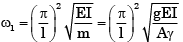QUESTION: 2

### A compacting machine shown in the figure below is used to create a desired thrust force by using a rack and pinion arrangement. The input gear is mounted on tile motor shaft. The gears have involute teeth of 2 mm module.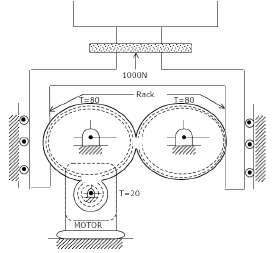Q.  If the drive efficiency is 80%, then torque required on the input shaft to create 1000 N output thrust is

Solution: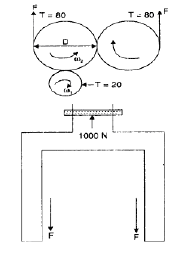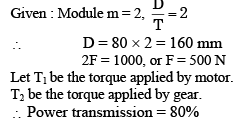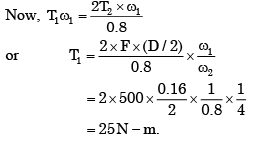QUESTION: 3

### A compacting machine shown in the figure below is used to create a desired thrust force by using a rack and pinion arrangement. The input gear is mounted on tile motor shaft. The gears have involute teeth of 2 mm module.Q.  If the pressure angle of the rack is 20°, then force acting along the line of action between the rack and the gear teeth is

Solution: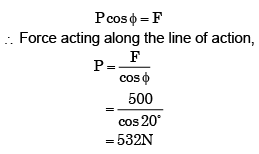QUESTION: 4

Assertion (A): The critical speed of an elastic shaft calculated by the Rayleigh's method is higher than the actual critical speed.

Reason (R): The higher critical speed is due to higher damping ratio.

Solution:
QUESTION: 5

An epicyclic gear train is shown schematically in the adjacent figure.

The sun gear 2 on the input shaft is a 20 teeth external gear. The planet gear 3 is a 40 teeth external gear. The ring gear 5 is a 100 teeth internal gear. The ring gear 5 is fixed and the gear 2 is rotating at 60 rpm (ccw = counter-clockwise and cw = clockwise).
The arm 4 attached to the output shaft will rotate at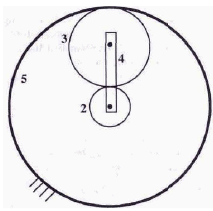Solution:
QUESTION: 6

The danger of breakage and vibration is maximum?

Solution:
QUESTION: 7

Two mating spur gears have 40 and 120 teeth respectively. The pinion rotates at 1200 rpm and transmits a torque of 20 Nm. The torque transmitted by the gear is

Solution: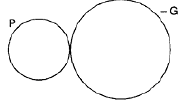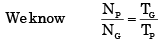where, NP speed of pinion, NG speed of gear wheel

TG = number of teeth of gear,
TP = number of teeth of pinion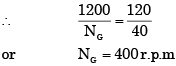Since power transmitted by both gear will be equal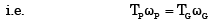where, TP = torque transmitted by pinion, TG = torque transmitted by gear wheelQUESTION: 8

A shaft of 50 mm diameter and 1 m length carries a disc which has mass eccentricity equal to 190 microns. The displacement of the shaft at a speed which is 90% of critical speed in microns is

Solution:
QUESTION: 9

The arm OA of an epicyclic gear train shown in figure revolves counter clockwise about O with an angular velocity of 4 rad/s. Both gears are of same size. The angular velocity of gear C, if the sun gear B is fixed, is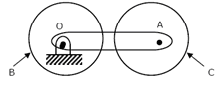Solution: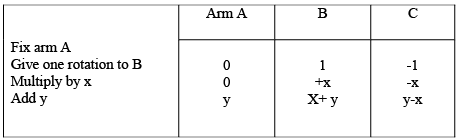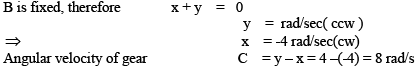QUESTION: 10

If a spring-mass-dashpot system is subjected to excitation by a constant harmonic force, then at resonance, its amplitude of vibration will be

Solution:
QUESTION: 11

Whirling speed of a shaft coincides with the natural frequency of its

Solution:
QUESTION: 12

The sun gear in the figure is driven clockwise at 100 rpm. The ring gear is held stationary. For the number of teeth shown on the gears, the arm rotates at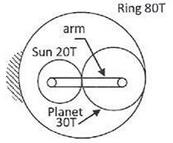Solution: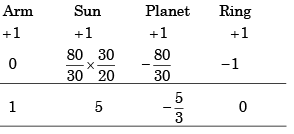For 5 Re volutions Of Sun , Arm rotates by 1
∴ for 100 revolutions of Sun, Arm rotates by 100/5 = 20

QUESTION: 13

Whirling speed of shaft is the speed at which

Solution:
QUESTION: 14

Large speed reductions (greater than 20) in one stage of a gear train are possible through

Solution:
QUESTION: 15

Consider the following statements

The critical speed of a shaft if affected by the
1. eccentricity of the shaft
2. span of the shaft
3. diameter of the shaft

Of these statements:

Solution:
QUESTION: 16

To make a worm drive reversible, it is necessary to increase

Solution:
QUESTION: 17

The rotor of a turbine is generally rotated at

Solution:
QUESTION: 18

For (ω1 = 60 rpm clockwise (cw) when looked from the left, what is the angular velocity of the carrier and its direction so that Gear 4 rotates in counter clockwise (ccw) direction at twice the angular velocity of Gear 1 when looked from the left?

Solution: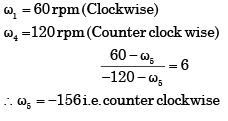QUESTION: 19

Assertion (A): Every rotating shaft has whirling speeds

Reason (R): Eccentricity of rotors on rotating shafts is unavoidable.

Solution: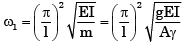QUESTION: 20

A 1.5 kW motor is running at 1440 rev/min. It is to be connected to a stirrer running at 36 rev /min. The gearing arrangement suitable for this application is

Solution:

speed reduction = 1440/36 = 40

QUESTION: 21

Consider the following statements:

When two gears are meshing, the clearance is given by the
1. Difference between dedendum of one gear and addendum of the mating gear.
2. Difference between total and the working depth of a gear tooth.
3. Distance between the bottom land of one gear and the top land of the mating gear.
4. Difference between the radii of the base circle and the dedendum circle.

Which of these statements are correct?

Solution:
QUESTION: 22

Assertion (A): When one body drives another by direct contact, their contact points must have equal components of velocity normal to the surfaces at the point of contact.

Reason (R): Two points in the same body must have the same component of velocity relative to the third body, in the direction of the line joining the two points.

Solution:
QUESTION: 23

The working surface above the pitch surface of the gear tooth is termed as

Solution:
QUESTION: 24

Match List I with List II and select the correct answer using the codes given below the lists: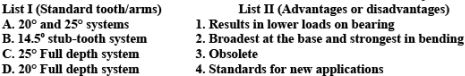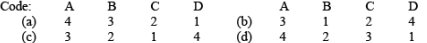Solution:
QUESTION: 25

Match List I with List II and select the correct answer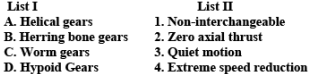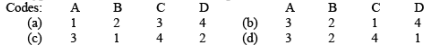Solution:
QUESTION: 26

Which type of gear is used for shaft axes having an offset?

Solution:
QUESTION: 27

When two shafts are neither parallel nor intersecting, power can be transmitted by using

Solution:
QUESTION: 28

Which one of the following pairs is not correctly matched?

Solution:
QUESTION: 29

The gears employed for connecting two non-intersecting and non-parallel, i.e., non coplanar shafts are

Solution:
QUESTION: 30

Mitre gears

Solution: